1.800.221.5175
Core Program
Full Access
Core Program
Full Access
High-Impact Tutoring
Core Program
Full Access
National Program
Full Access
New York
Critical Thinking for Active Math Minds
Preparing for Standards Based Assessments
Vocabulary Workshop, Tools for Comprehension Grades 1–5
Print Program
Interactive Edition
Print Program
Interactive Edition
Vocabulary Workshop, Tools for Excellence Grades 6–12+
Print Program
Interactive Edition
Vocabulary for Success
Progress English Language Arts Grades K–8
National Program
Full Access
High-Impact Tutoring
New York
Grammar Workshop, Tools for Writing
Grammar for Writing
Writing a Research Paper
Writing Workshop
Print Program
Interactive Practice Bundle
Fluency Booster Practice Book
Interactive Assessments
High-Impact Tutoring
Building Reading Success with Wiley Blevins
Print Program
Interactive Edition
Professional Learning

## How Math Games and Activities Increase Student Proficiency in Grade 7

Math games and activities can be an excellent tool to supplement and support math instruction. Using math games in your classroom allow students to practice mathematics in fun but also impactful ways. Students love games because they are engaging and exciting, and teachers love games because they help students practice what they've learned.

Grade 7 students develop their skills to reason abstractly and quantitatively and learn the importance of using representative samples for drawing inferences. They study ratios, scale drawings, rational and negative numbers, and how to graph proportional relationships. Solving real-world problems, (including objects such as triangles, quadrilaterals, and polygons) and practicing how to calculate interest and discounts are aided by activities that use a variety of models.

## Distributed Fraction Model

for Decimals

### Physical and visual models of decimals are difficult to extend for more than a few places, because the size of the model changes so drastically as you move from place to place. Viewing decimals as a sum of fractions gives students another way to think of decimals.

On the board, write 237.9036 in standard decimal form and as a sum of products.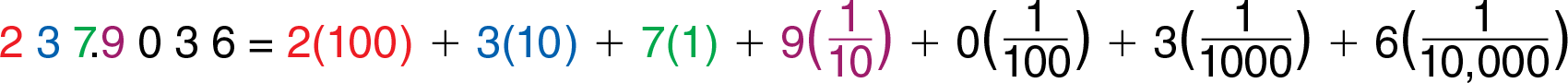Next, draw a number line like the one shown below. Use an arrow to represent each digit of the number written on the board. As you “build up” the number, students can see how relatively little the digit in each additional decimal place affects the size of the decimal.

Start with the first two digits: 2 3 _ . _ _ _ _Note that even if the tens digit were 9 (the highest possible), the number would not reach 300. Write the next digit and draw its arrow: 2 3 7 . _ _ _ _Note that even if the ones digit were 9 (the highest possible), the number would not reach 240. Continue with the next digit: 2 3 7 . 9 _ _ _Note that even though the tenths is 9 (the highest possible), the number does not reach 238.

Discuss the fact that no matter how large each succeeding digit is, it’s arrow cannot be longer than the arrow before it.

#### Inquiry Strategies

1. Understand Ask, “If I kept drawing arrows, how long would the arrow for the “3” in the thousandths place be? How would it compare with the arrow for the “3” in the tens place?” It would be too short to see. It would take 10,000 of them end-to-end to equal the length of the arrow for the “3” in the tens place.
2. Evaluate On the board, write 1234 and 0.1234. Ask, "In each number, how does the combined value of the last three digits compare to the value of the first digit?" In both cases, the combined value of the digits 2, 3, and 4 is less than 14 the value of the first digit.

## Area and Arrow Models

for Dividing by Fractions

### On the board, write 8 • 3⁄4 . Then, as outlined below, use a pictorial or physical area model to show that multiplying by 3⁄4  is the same as multiplying by 3 and then dividing by 4.Since the fraction bar represents division, another way to think about 34  is 3 ÷ 4.Think of multiplying by the fraction 34  as two operations with integers:Since division is the inverse, or opposite, of multiplication, you can divide by using the opposite operations as we used for multiplying.

Think of dividing by the fraction 34  as two operations with integers:Since multiplying by 4 and then dividing by 3 is the same as multiplying by 43, to divide by 34 , you can multiply by 43 .

#### Inquiry Strategies

1. Analyze Have students look again at the last step and note the order: multiply then divide. Ask, “How come the order of the two operations does not affect the result?” If students think of dividing by 3 as multiplying by 13 , they can cite the commutative property of multiplication.
2. Apply Ask, “When one fraction is divided by a different fraction, does it matter which fraction is changed to its reciprocal? Use an example to explain.” Yes, it matters because division is not commutative. For example, consider 12 ÷ 14 .  Changing 12  to  21  and multiplying does not give the correct quotient:  21  •  14  =  24  , or  12 .  Changing  14  to  41  and multiplying gives the correct quotient:   12  •  41  =  42  , or 2 .

## Numberless Staircase Model

for Slope

### To focus students’ attention on how the behavior of a function is reflected in the shape of its graph, use a model that does not involve numbers and represents the different types of slopes.

Compare these models, each with a different kind of step, or slope, as shown below. Emphasize that slope is a ratio that relates the vertical change, rise, or y, to the horizontal change, run, or x. Note that the three models at the top show a positive rise from left to right, reflecting a positive change in y.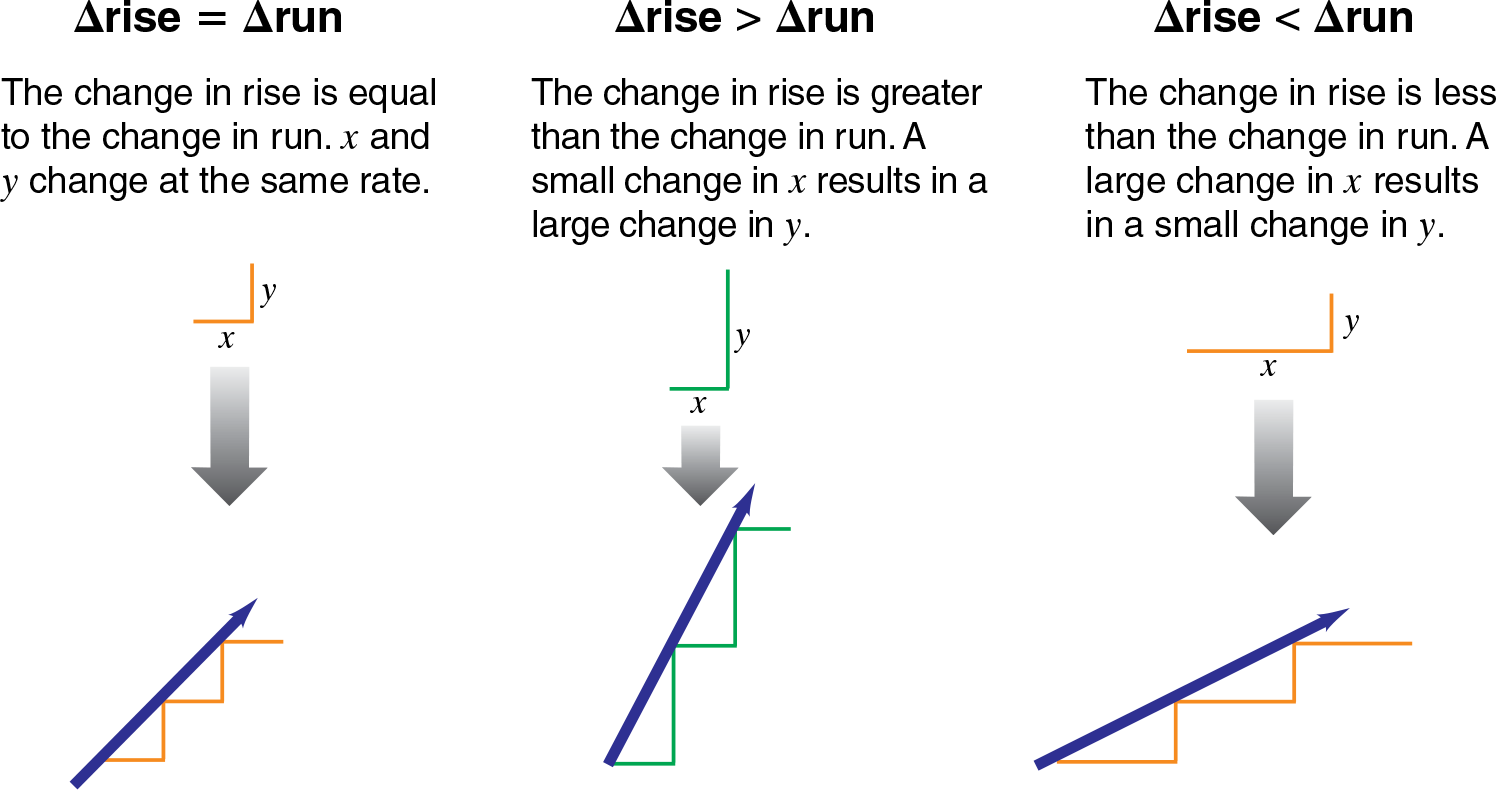Use the same approach for models that show functions that “fall” from left to right, representing a negative slope in which the change in y is negative.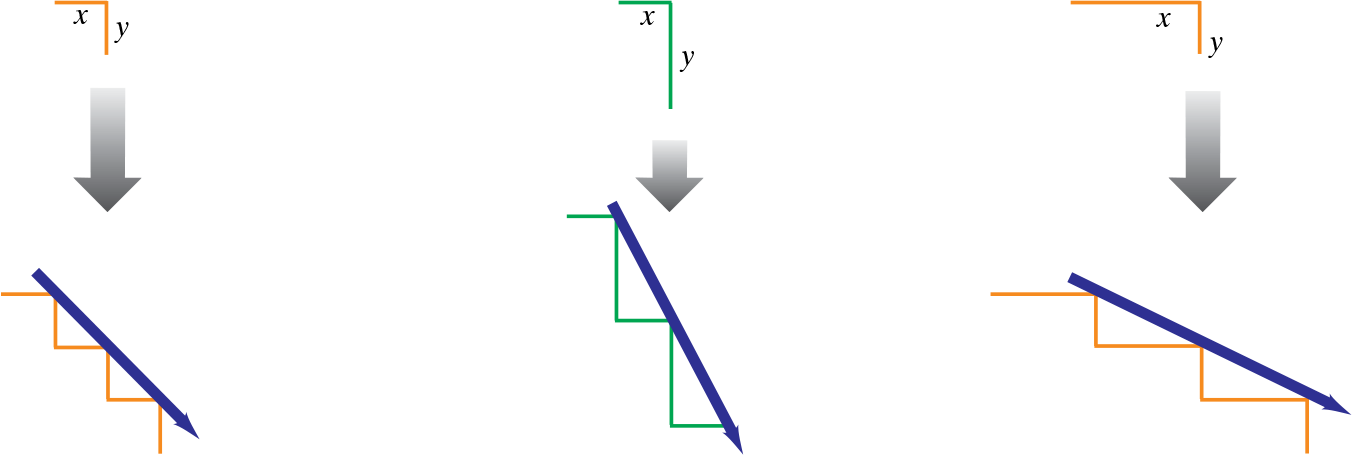#### Inquiry Strategies

1. Understand Say, “Imagine a function represented by the model at the right in the top row. If x increases from 3 to 7, what would be the approximate change in y? “Can you tell what the beginning and ending value of y would be? Why or why not?” About half of 4, or 2; No. The model does not show x and y values, just the relative changes in x and y; however, you would be able to tell if the function is graphed on a coordinate plane.
2. Apply Explain that a store is selling its used CDs for \$2.25 each. Ask, “Which of the 6 models above best represents the dependence of the total cost on the number of CDs bought? Explain.” The model in the middle of the top row; for each unit to the right, the run—the number of CDs bought—the rise, or dollar increase, is about 2.25 units. The change in the rise is greater than the change in the run.

## Bar Graph Model

for Length-Area-Volume

### Construct a graphic display to show the relationships among length, area, and volume.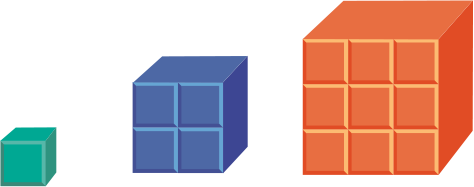1. Start with a unit cube. Construct two other cubes, one whose edge length is twice, and another whose edge length is three times that of the original cube.

2. Disassemble each larger cube into unit squares, and stack each group to establish a bar that shows the total number of unit squares and cubes. This creates a triple-bar graph as shown below.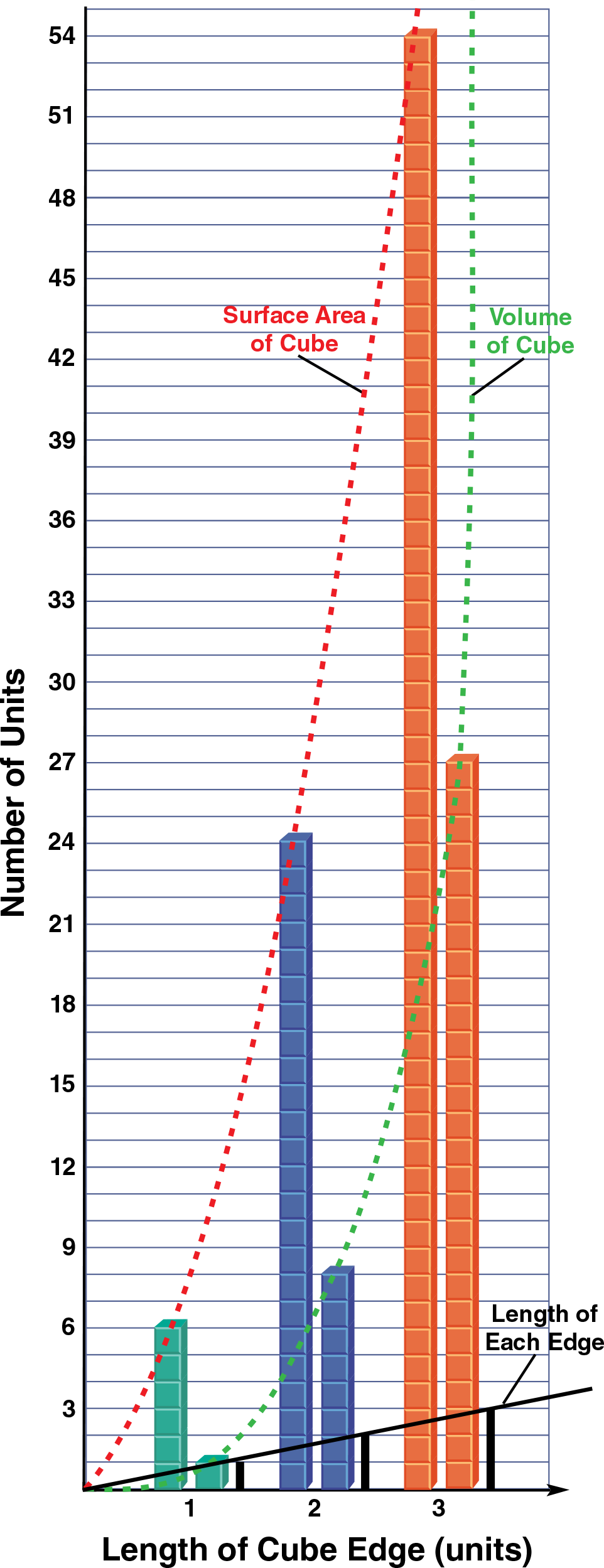3. Using a different color for each, draw a line or curve through the top of the length of each edge bars, the top of the area bars, and the top of the volume bars.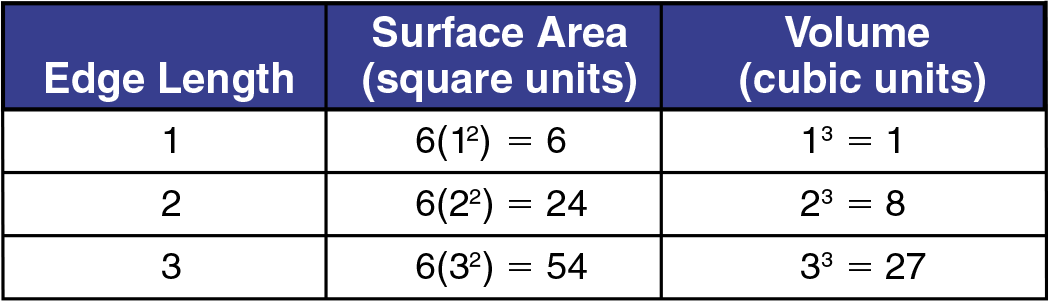#### Inquiry Strategies

1. Evaluate Ask, “If you make each edge of a cube 10 times as long, what will happen to the surface area, and what will happen to the volume? The surface area will be 100 times as great, and the volume will be 1000 times as great.
2. Apply Ask, "If a puppy grows twice as tall in a year, what do you expect would happen to its weight? Why?" The puppy would weigh about 8 times as much because its volume would be about 8 times as great.

## Bar Graph Model

for Mean and Median

### Use a bar graph of data to illustrate the difference between mean and median.

• Start with a graph like the one below, showing goals scored in 11 soccer games.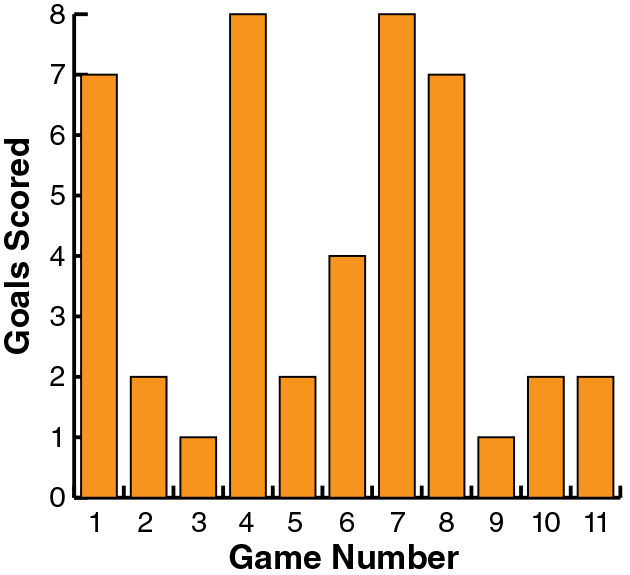• Then show the data in order from least to greatest. On the rearranged graph, display the median and the mean as shown.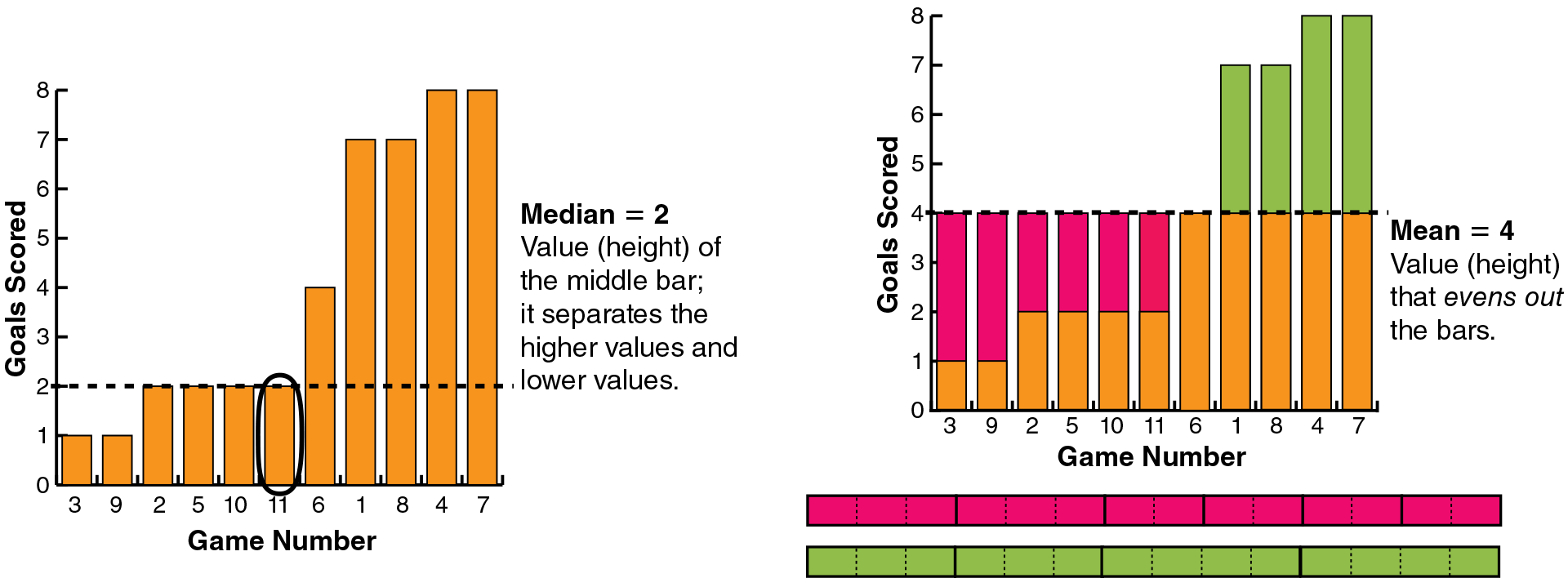#### Inquiry Strategies

1. Analyze Ask, "Why is the mean of this data greater than the median?" Discuss how each measure describes the average score. Students should see that the four high-scoring games bring up the mean but not the median. If two fewer goals were scored in each of those high-scoring games, the median would stay the same but the mean would be less.
2. Evaluate Write or just say the following statement: In general, if you find how far each data value is from the mean, the total of the differences above the mean equals the total below. Have students use the model to decide whether the statement is true and why. The statement is true. On the model, the totals of the differences are indicated by the red and green areas. The green area above the mean equals the red area below the mean.

## Visual Model

for Multiplying Polynomials

### The displays below show how the partial products generated from multiplying polynomials are analogous to those generated from multiplying numbers.

Example with Numbers: Multiply 4 × 357.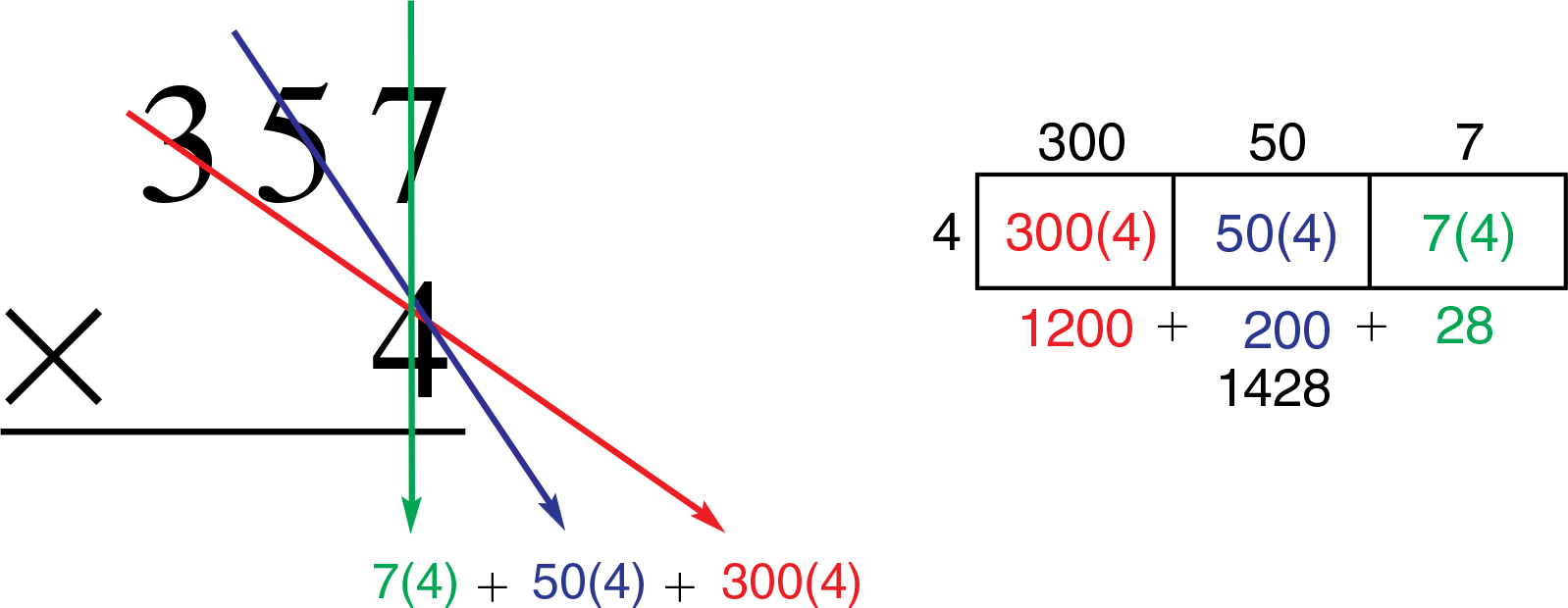Example with Polynomials: Multiply 3x (x2 + 2x − 6)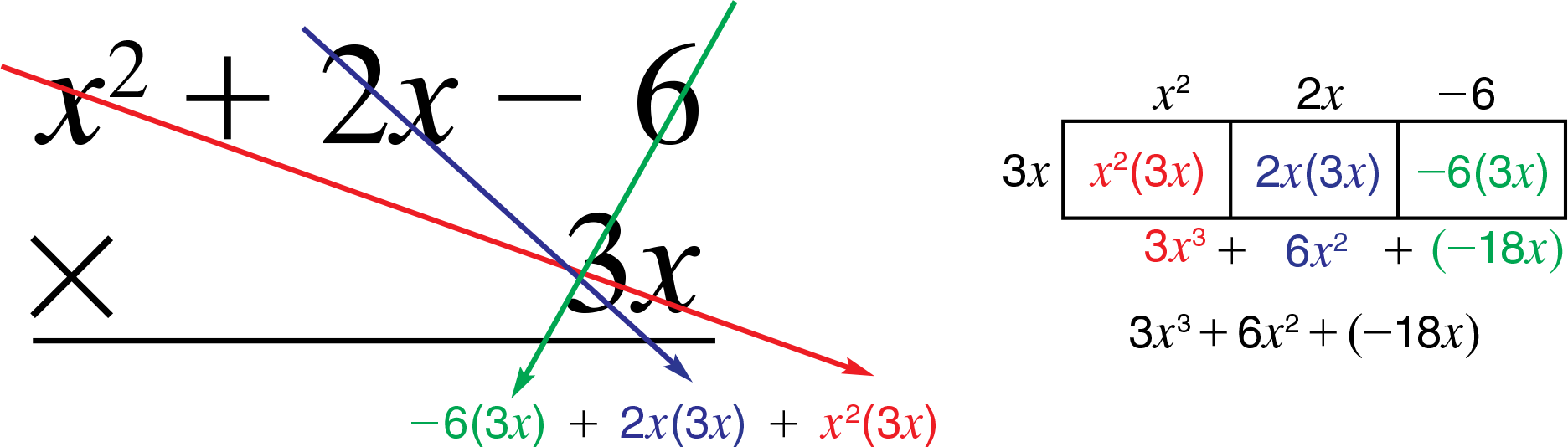#### Inquiry Strategies

1. Analyze On the board, write:Ask, “How does writing 4 × 357 in this way help you explain why multiplying a trinomial by a monomial is like multiplying a 3-digit number by a 1-digit number? Responses may indicate that decomposing 357 into hundreds, tens, and ones makes it easier to see the connection between the two examples.
2. Apply Write 5b (a + b + c) on the board. Ask, “Will this product contain the term 5ab2c? Why or why not?” No. The term 5b is multiplied by a, by b, and by c, resulting in 3 unlike terms, none of which contains all 3 variables a, b, and c.

## Bar Model

for Percent Change

### Use visual models and cutouts to reinforce how a percent increase or decrease describes the size of a change in relation to the original amount.Use a similar procedure to model a percent decrease of 25%.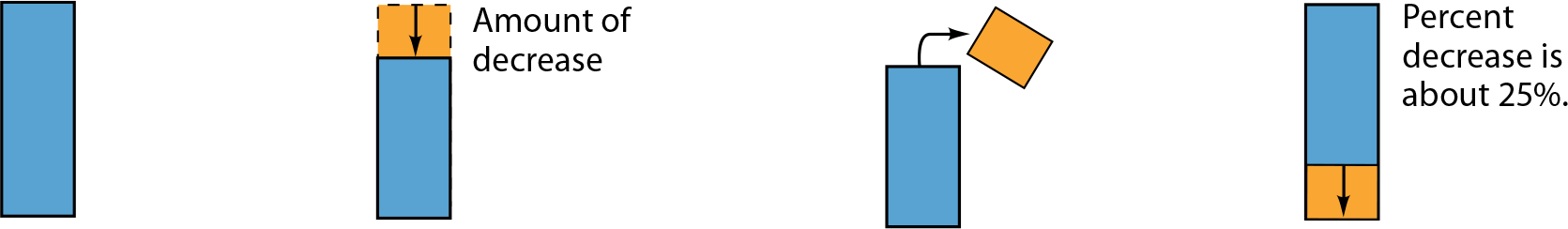#### Inquiry Strategies

1. Apply Ask, “What is the percent increase if the price of a ticket to a baseball game doubles? What if it triples? Explain.” When something doubles, the amount of increase is equal to the original amount, so the percent increase is 100%. When something triples, the increase is twice the original amount, so the percent increase is 200%.
2. Analyze Have students explain how the two statements below are different. In each case, ask them to give the percent increase.
1. The price of gasoline this week is 120% of last week’s price.
2. The price of gasoline has gone up 120% since last week.
1. Statement A compares the new price to the old price, statement B compares the change to the old price. Statement A describes a 20% increase, while B describes a 120% increase–6 times as much as the increase in the first statement!

## Bar Graph Model

for Perimeter-Area Relationship

### Build a graph to show the relationships among side length, perimeter, and area.

1. Start with a square and construct three other, larger squares with side lengths two, three, and four times the length of the side of the original square.

2. Disassemble each larger square into unit squares and stack the unit squares to establish a bar graph.

3 Draw a line next to each stack of unit squares to show the total side length, or perimeter, of each constructed square.

4. Draw a line through the tops of the perimeter bars and a curve through the tops of the area bars.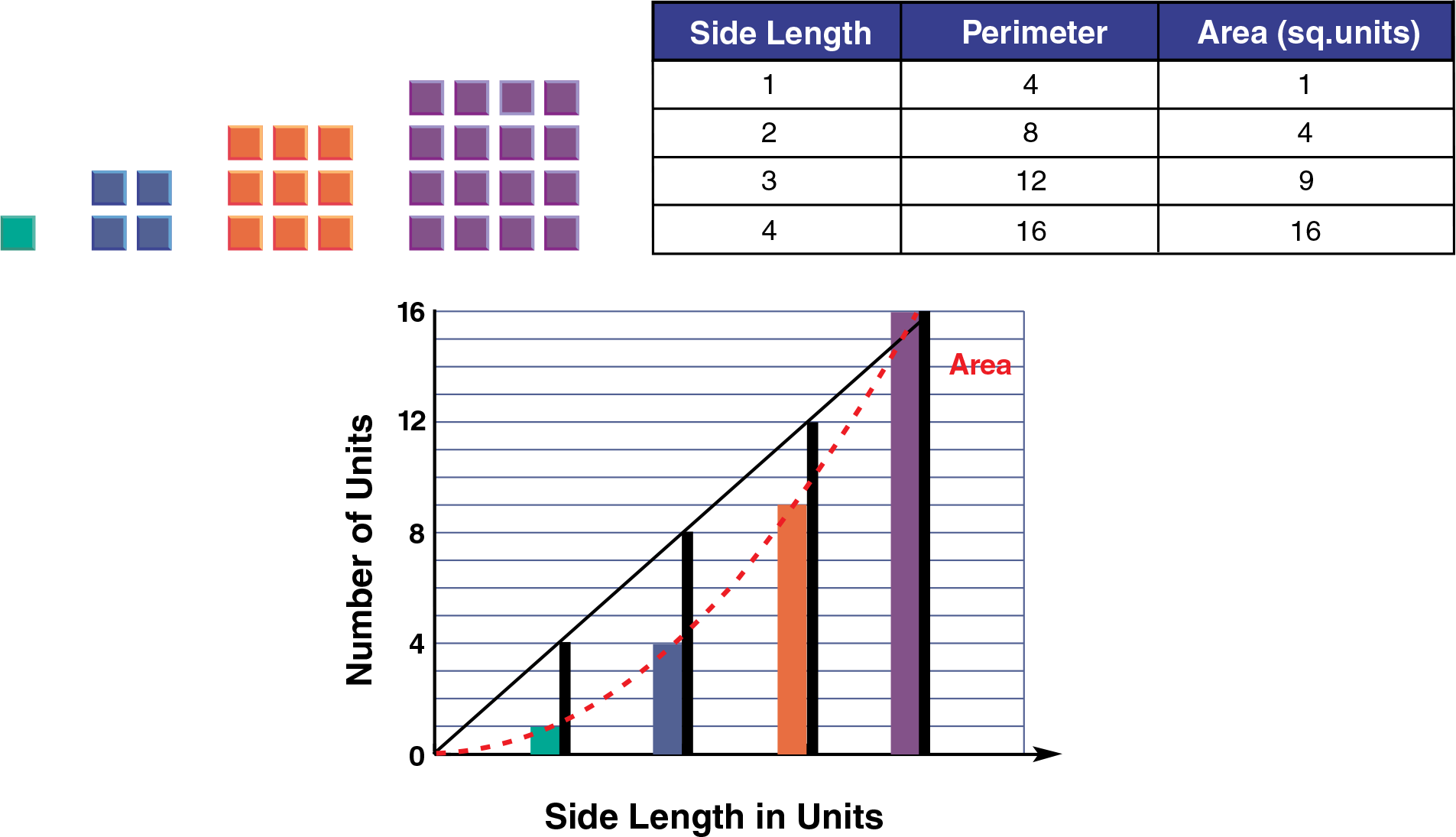#### Inquiry Strategies

1. Apply Ask, “If you make each side of a square 10 times as long, what will happen to the perimeter, and what will happen to the area? The perimeter will be 10 times greater and the area will be 100 times greater.
2. Evaluate Ask, “If you double the dimensions of a triangle, what will happen to the area? Why? The area quadruples. Possible explanation: Both the base and height are doubled. When you multiply them to find the area, you get an area four times greater.

## Area Model

for Probability

### Use a rectangle to show why the product of 1⁄6 × 1⁄6 can be used to find the probability of rolling a 5 and then another 5 when a 1–6 number cube is rolled twice.

1. Divide a rectangle into 6 equal sections to show the number of times you would expect each number on a 1–6 number cube to come up when the cube is rolled many times.
Shade the "5" section. Express the number as a fraction: 16.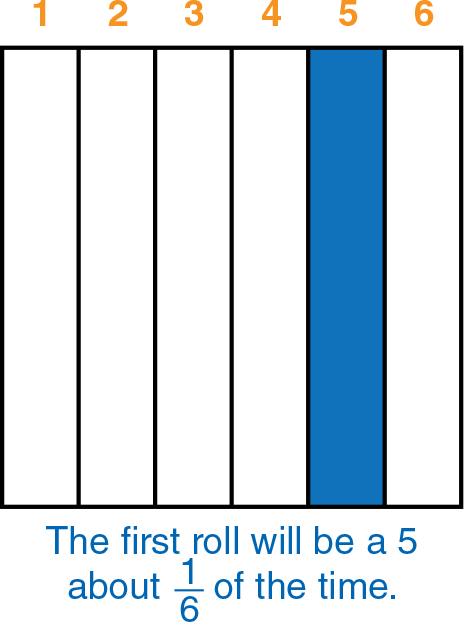2. Divide the “5” section into 6 equal subsections to show the number of times rolling a 5 will be followed by a roll of each number.
Shade the “5” subsection. Express the number as a fraction: 16 . Multiply the two fractions.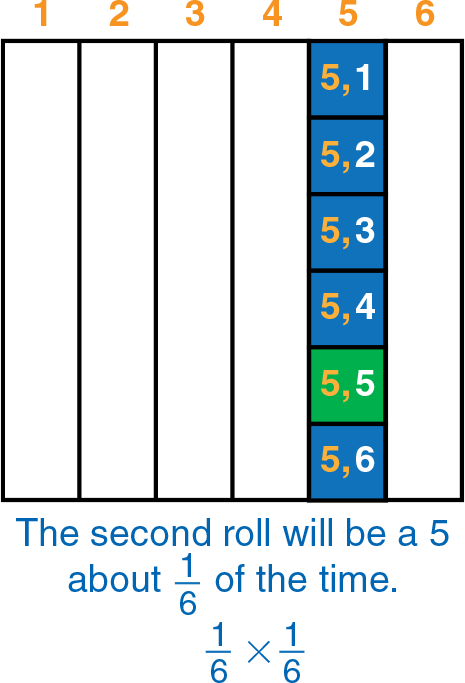#### Inquiry Strategies

1. Understand Call students' attention to the dark-shaded subsections in the "5" section of the rectangle above the right. Ask, "What would the bottom subsection represent? Why are the 6 subsections equal?" The fraction of times you would expect to roll first a 5 and then a 6; On any roll, each number has an equal chance of coming up.
2. Create Have students use a circle to make an area model for the probability of tossing three heads when tossing a fair coin. Models should look something like those below.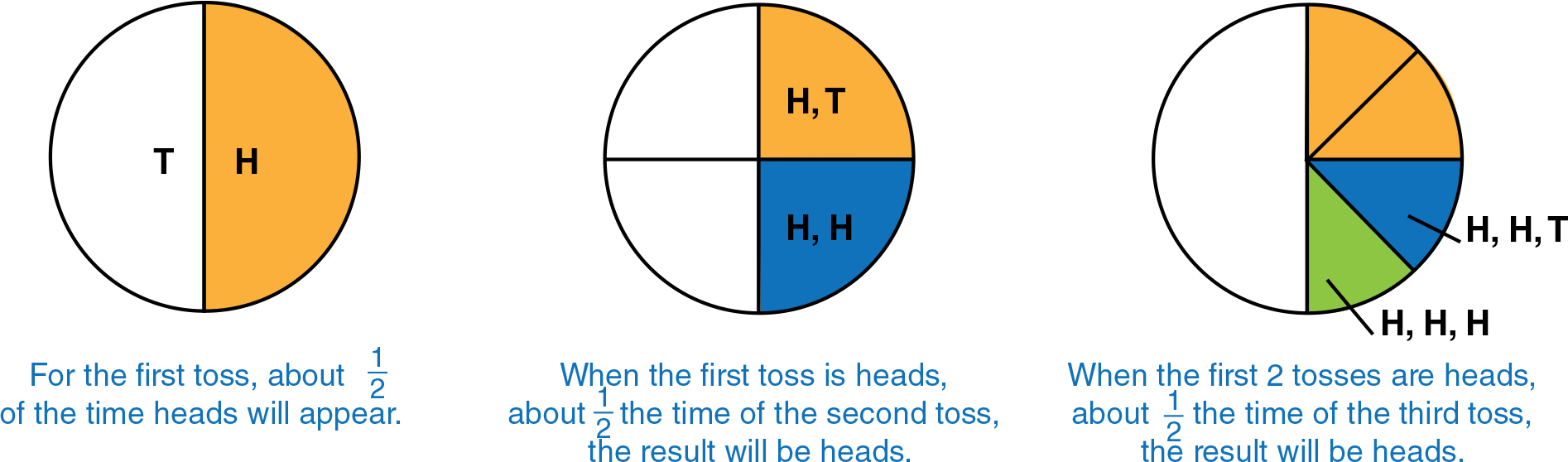## Geometric Models

for Proportions

### A geometric model for proportions takes advantage of students’ familiarity with analogies and other patterns that involve geometric figures.

• Place 3 physical models or drawings of geometric figures in a standard proportion format. Have students place, draw, or describe the figure that belongs in the blank space.• Ask a volunteer to rearrange the figures to create another visual proportion.
Possibilities include:• To relate this visual model more closely to numerical proportions, use squares and triangles divided into congruent parts.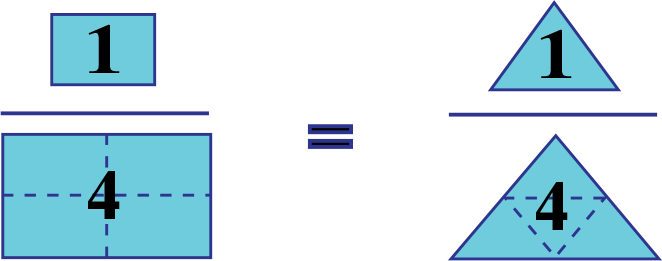#### Inquiry Strategies

1. Understand Display these two visual ratios with a question mark between them. Ask, “Is this a true proportion? Why or why not?” No, the ratios are not the same. One shows a relationship of ‘small to large,’ and the other a relationship of ‘large to small.’
2. Evaluate Ask, “When a photograph is enlarged proportionally, what ratio stays the same?” Any ratios of two corresponding distances, such as the ratio of length to width.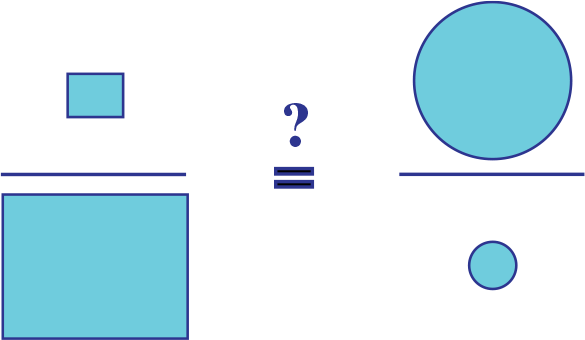## Dynamic Model

for Related Angles

### Use physical models to show how angles can change while the relationship between them is constant.

Model for Angles of a Transversal

Cut out 3 strips of cardboard and assemble them with fasteners to form a transversal. Rotate the transversal as shown (the other strips remain parallel).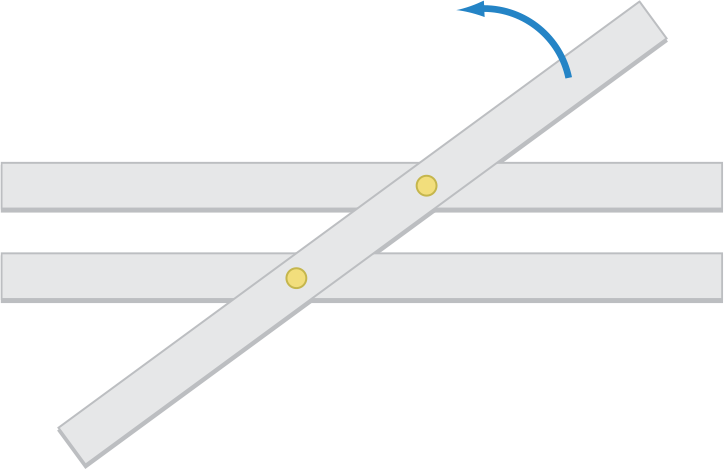As the angles change in size, focus students’ attention on pairs of angles that remain congruent and pairs that remain supplementary.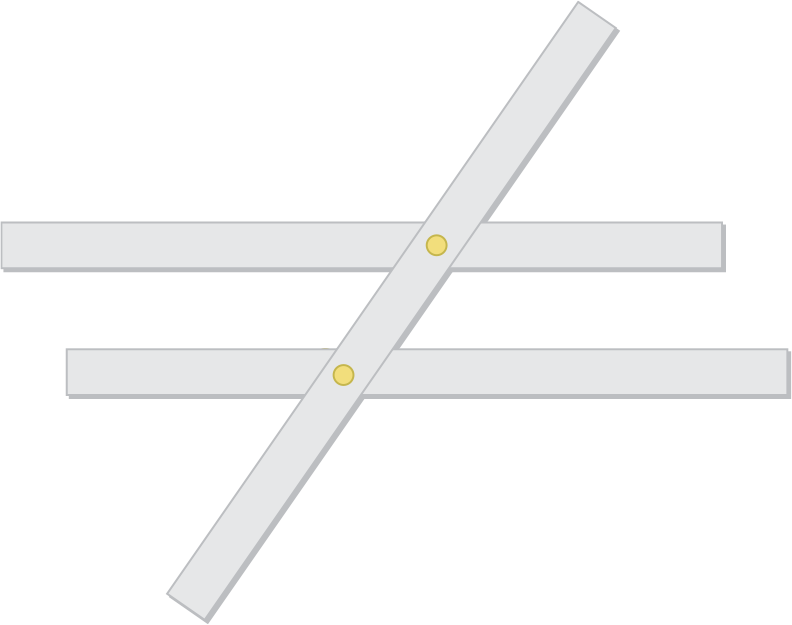Model for Angles of a Parallelogram

Use 4 strips and fasteners to form a rectangle. Rotate a pair of opposite sides as shown . . .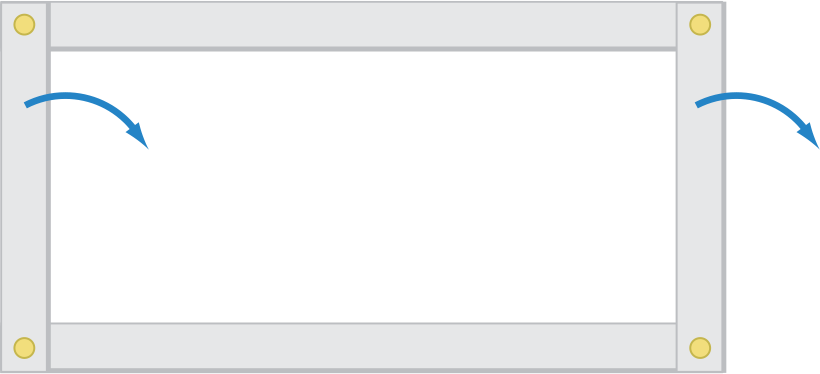... to create a nonrectangular parallelogram. As the angles change in size, focus students’ attention on pairs of angles that remain supplementary and are congruent. Point out how the decrease in one angle equals the increase in another angle.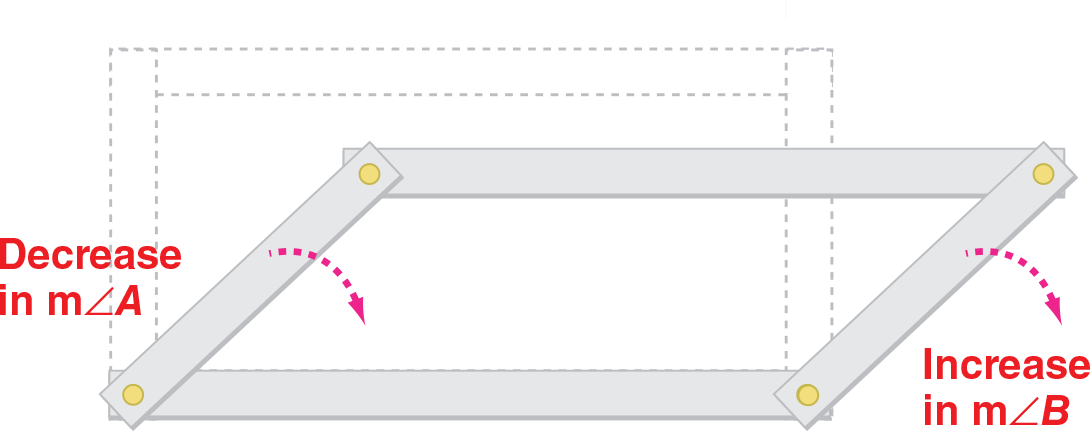#### Inquiry Strategies

1. Create Have students draw a diagram of a transversal intersecting three parallel lines to form 12 nonright angles. Ask students to label sets of angles that are always congruent. Students should label all the obtuse angles congruent as well as all the acute angles.
2. Analyze Call attention to the parallelogram model. Point out that as the measure of angle A decreases, the measure of angle B increases by the same amount. Ask, “What does that tell you about the total of the measure of the 4 interior angles of a parallelogram?” The total measure of the 4 angles must be 360°, the same as the total for a rectangle, because any decrease in one angle is offset by an equivalent increase in another.

## Balance Model

for Solving Equations

### Objects placed on the pans of a balance push down with the force of their weight. So, positive quantities and addition can be represented on a balance model simply by placing objects or drawing figures on the pans. Negative numbers and subtraction can be represented by the opposite of a downward force, an upward force – such as the force of someone pushing up from under one of the pans.

Example: Solve 3x − 5 = 7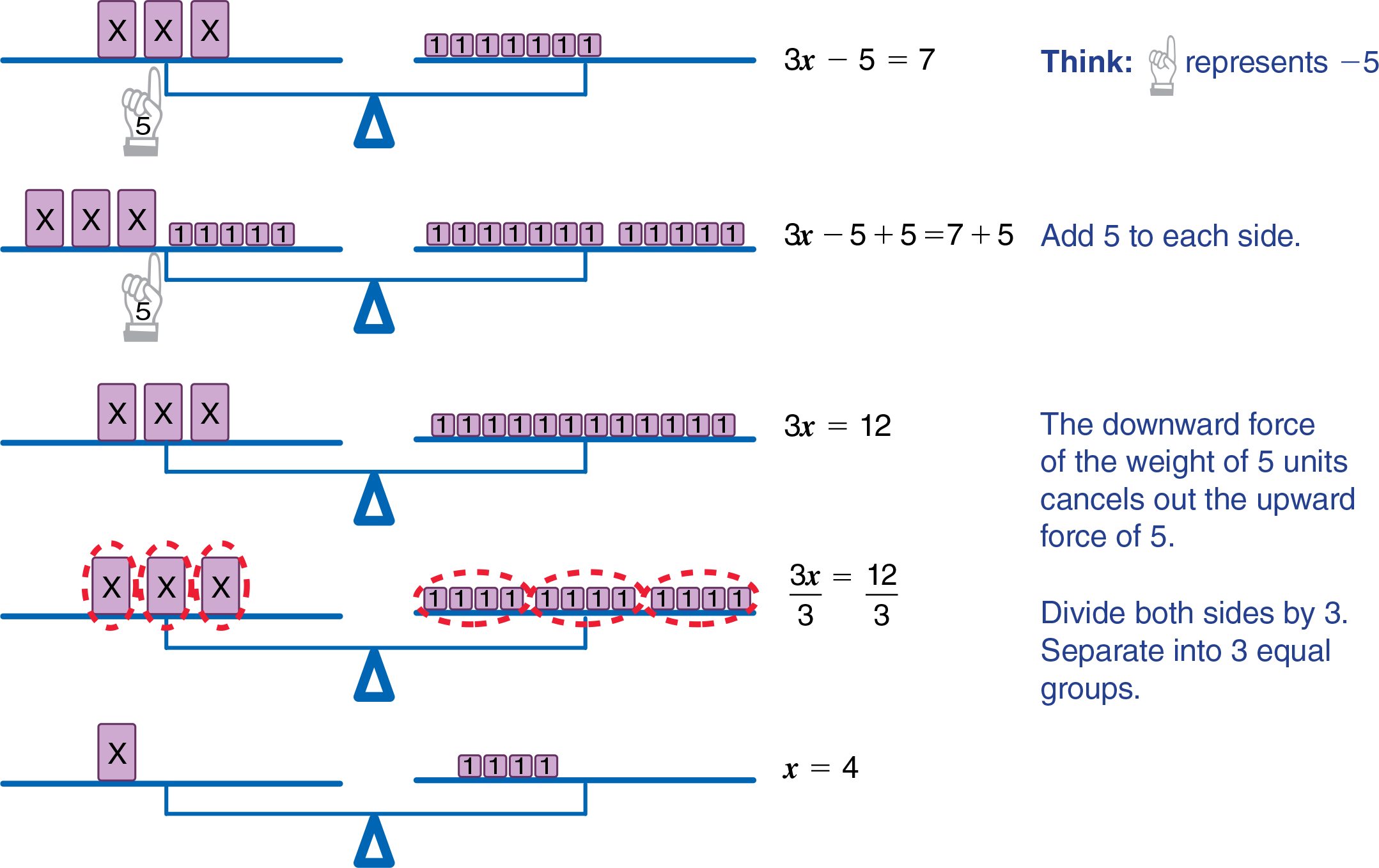#### Inquiry Strategies

1. Evaluate Ask, “Why does it make sense to show a negative number as a force pushing up?” A negative quantity is the opposite of the corresponding positive number; a force pushing up is the opposite of a force pushing down.
2. Apply On the board, write 2x + 3= 7. Ask, “How would you use the balance model to solve this equation?” Left pan has 2 boxes marked “x” and 3 unit boxes. Right pan has nothing on it, but a hand underneath it is pushing up with a force of 7.

## Number Line Model

for Solving Inequalities

### An inequality like 4 < 6 can be pictured on a number line. Given two real numbers, the lesser number will always be to the left on the number line.

Show two numbers on a number line and provide examples of adding the same amount to the two numbers.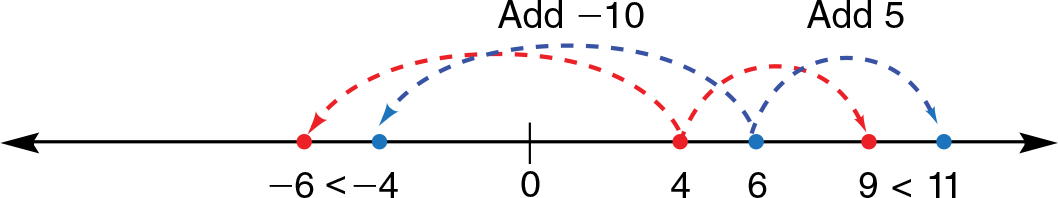Note that in every case, the sums are still in the same order. The red point is still to the left of the blue point.

Use a similar model to show that subtracting the same amount from the original numbers does not change the order either.

Then use the model to demonstrate what happens when two numbers are multiplied (or divided) by the same positive amount. Again the order does not change.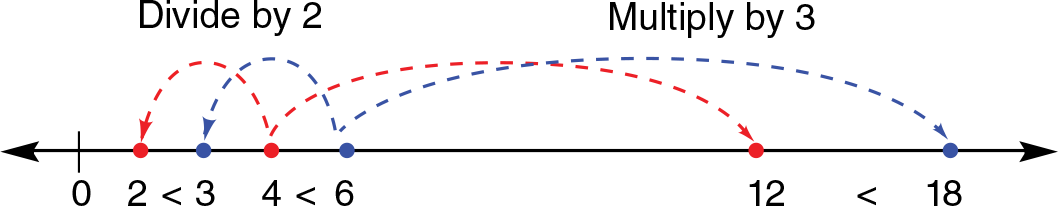Finally, use the model to show that multiplying (or dividing) by a negative amount does not change the order. Note that the red and blue points switch places. The inequality sign must be reversed to keep the sentence true.#### Inquiry Strategies

1. Understand Ask, “How would you explain why the inequality sign must be reversed when you multiply both sides of an inequality by a negative number but not when you add a negative number to both sides of an inequality?” Students may use a number line to show that multiplying by a negative number switches the order of the products on the number line.
2. Apply On the board, write –3x < 6. Ask, “If you divide both sides by 3, does the relation sign need to be reversed?” “What if, in the next step, you multiply both sides by –1?” no; yes.

## Coin and Counter Models

for Negative Numbers

### Models can help students understand integers below zero, but no model seems to work perfectly in all instances1. Coins or two–colored counters are particularly useful for helping students understand the distinction between negative quantities and subtraction.

Let one side of a coin or counter represent 1 and the other side -1.Use the model to show the different meanings of the minus sign in a numerical expression.

Example: Simplify −|−6 − (−2)|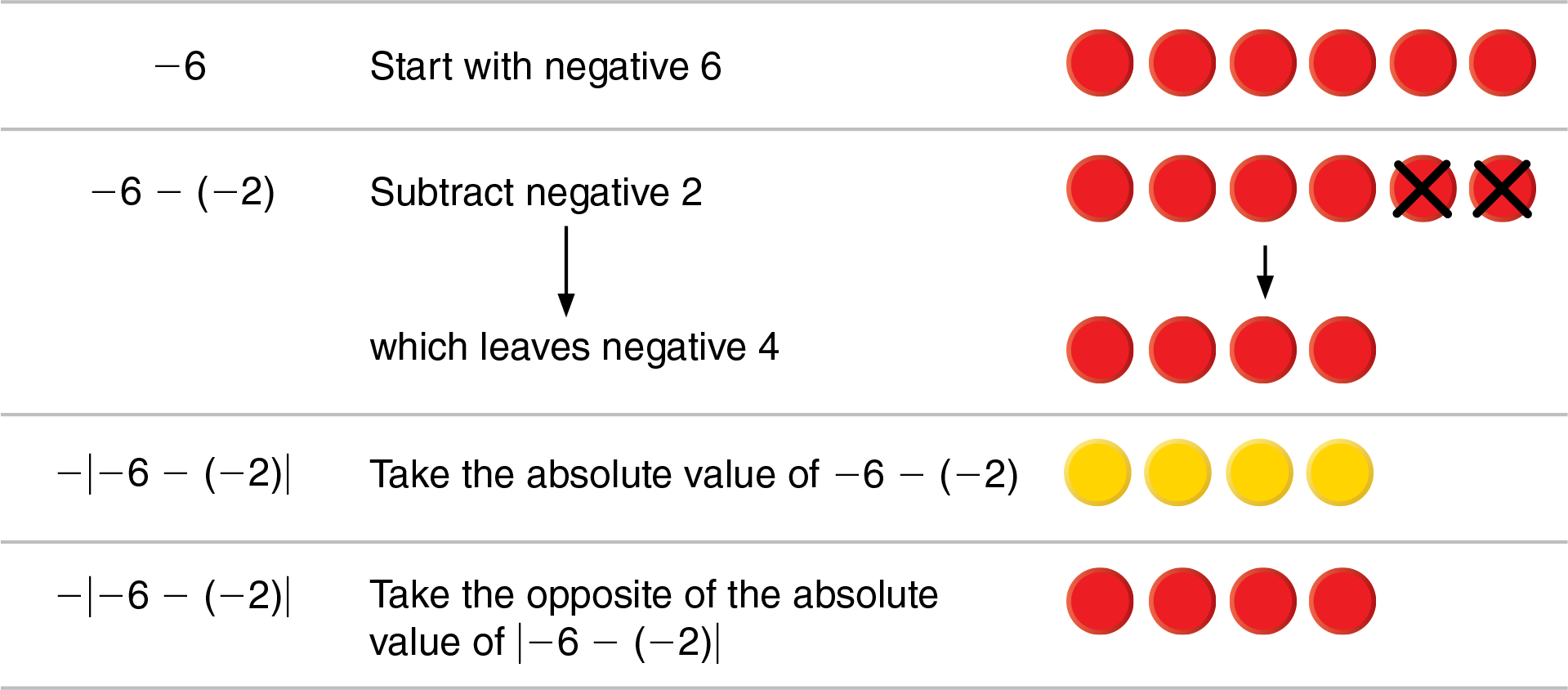So, −|−6 − (−2)| = −4.

#### Inquiry Strategies

1. Summarize Ask, “What are the two ways of using the minus sign, and how are they different?” Students should recognize the distinction between subtracting, which means taking away or finding the difference and taking the opposite of, which means finding the additive inverse.
2. Analyze On the board, write −(−5 − 5). Ask, “In this expression, what is the meaning of each minus sign?” Have students simplify the expression. The first means take the opposite of −(−5 − 5) . The second means take the opposite of 5. The third means subtract 5. The result is 10.

1 Fuson, Wearne, Hiebert, Murray, Human, Olivier, Carpenter, and Fennema, 1997; Hiebert, Carpenter, Fennema, Fuson, Wearne, Murray, Olivier, and Human, 1997 Cited in Adding it Up